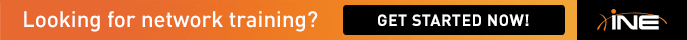# T2.5 Reported Distance Problem

Reffering to the EIGRP concept, the Unequal-Load Balancing are going to occur, if the secondary path has lower reported distance than the primary path. In this case, if R4 want to reach 222.22.2.0/24 it have 2 possible routes, that is directly over R5 which will have RD=x and via R1 thath will have RD=x+y

in this case, eventhough we modified the metric, doesn't mean that we will overcome this issueRack8R4#siet 222.22.2.0
IP-EIGRP (AS 1024): Topology entry for 222.22.2.0/24
State is Passive, Query origin flag is 1, 1 Successor(s), FD is 2560000512
Routing Descriptor Blocks:
174.8.45.5 (FastEthernet0/1), from 174.8.45.5, Send flag is 0x0
Composite metric is (2561536256/2560000256), Route is External <--
Vector metric:
Minimum bandwidth is 1 Kbit
Total delay is 60010 microseconds
Reliability is 1/255
Minimum MTU is 1
Hop count is 1
External data:
Originating router is 150.8.5.5
AS number of route is 0
External protocol is RIP, external metric is 7
174.8.145.1 (Serial0/0), from 174.8.145.1, Send flag is 0x0
Composite metric is (2565504512/2560000512), Route is External <--
Vector metric:
Minimum bandwidth is 1 Kbit
Total delay is 215020 microseconds
Reliability is 1/255
Minimum MTU is 1
Hop count is 2
External data:
Originating router is 150.8.5.5
AS number of route is 0
External protocol is RIP, external metric is 7

we see that if R4 using F0/1(R4-R5-destination) the RD=2560000256, while using S0/0(R4-R1-R5-Destination) the RD=2560000512

so I think that this task cannot be solved unless we have another way to trick the EIGRP not to compare the RD in the process of Unequall-Load cost balancing

any other idea?!?

RD must be lower then current Succesor, in this case, this unqual-load balancing is possible in this scenario